## Statistics 2MA3 - Test #3 Solutions

### 2002-03-21

The marking scheme is indicated in red. Full Marks = 50.

#### Q1

(a) Define the following terms: heteroscedasticity, robustness, P-value, type I error, type II error. [5 marks]

Heteroscedasticity means that the variance of a random variable is not the same in each subpopulation or at all levels of the covariates.

A statistical method is said to be robust if it does what it is supposed to do even when the assumptions on which it is based are not satisfied.

There are three definitions of P-value; any one is acceptable here. (Definition 1) P-value is the smallest level of significance that will lead to rejection of the null hypothesis with the given data. (Definition 2) P-value is the largest level of significance that will lead to acceptance of the null hypothesis with the given data.(Definition 3) P-value is the probability of getting a value of the test statistics as extreme as, or more extreme than, the value observed, if the null hypothesis were true. The alternative hypothesis determines the direction of "extreme".

Rejecting the null hypothesis when it is true is called a type I error. Failing to reject the null hypothesis when it is false is called a type II error.

(b) What pivotal quantity is used to compare two variances? Under what assumptions is it valid? Write out the confidence interval formula derived from it, using Rosner's notation for the quantiles of the F distribution. [7 marks]

Given two different populations, the pivotal quantity for the ratio of population variances q, given the ratio of sample variances, and the corresponding confidence interval, are given by

 Pivotal Quantity Confidence Interval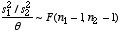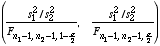Assumptions: the two samples are independent of each other and the observations in each sample are independent and normally distributed.

(c) Give 5 interesting facts about William Sealy Gosset. [3 marks]

William Gosset was born on 13 June 1876 in Canterbury, England and died on 16 October 1937 in Beaconsfield, England. He was educated at Winchester, then entered New College, Oxford where he studied chemistry and mathematics.

Gosset was an applied chemist. He used a combination of mathematics and empirical studies with random numbers to guess the formula for the t-distribution and he got it right.

Gosset was employed by the Guinness brewery in Dublin, Ireland. Guinness did not want him publishing in his own name, for fear of revealing trade secrets, so he published his work on the t-distribution under the pseudonym of "Student".

Gosset was using a haemocytometer to count yeast cells at the brewery and he used the Poisson distribution to model the variation between observations.

At the end of 1935 Gosset left Ireland to take charge of the new Guinness brewery in London.

#### Q3

Analyse the following two data sets with appropriate graphics and P-values. State your assumptions and your conclusions. Where possible, assess the validity of your assumptions. [30 marks]

(a) Students randomly assigned 22 giant freshwater prawns to two different aquaria and recorded their weight in grams after 25 days.

The analysis is difference of means for two independent samples, assuming independence, normality, homoscedasticity.

Graphical analysis: comparative dot plot or comparative box plot.

• Independence: can't test; as the prawns aren't in any serial order, testing for autocorrelation won't be possible.
• Normality: could draw histograms or stem-leaf plots but the comparative dot plot or comparative box plot (shown) is enough to indicate that the two distributions are reasonably symmetric and free of outliers.
• Homoscedasticity: do a test for the ratio of variances.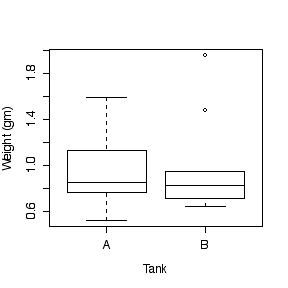P-value to test the hypothesis that the difference in means is zero:

```> xbara <- mean(weight[tank=="A"])
> xbarb <- mean(weight[tank=="B"])
> s2a <- var(weight[tank=="A"])
> s2b <- var(weight[tank=="B"])
> xbara
 0.94
> xbarb
 0.9545455
> s2a
 0.0896
> s2b
 0.1603673
> s2p <- (10*s2a + 10*s2b)/20
> s2p
 0.1249836
> t0 <- (xbara-xbarb)/sqrt(s2p*(1/11 + 1/11))
> t0
 -0.09648995
> 2*pt(t0,20)
 0.9240918
```

Since t0 = -0.096 is in the left tail of the t distribution on 20 degrees of freedom, using the tables in Rosner we find that |t0| = 0.096 < t20,0.75 = 0.687 so we have that P > 2*(0.25) = 0.5. (The exact value is P = 0.924.)

Because the P-value is large, we conclude that there is no evidence from these data that mean prawn growth rate varies between the two tanks.

F-test for the ratio of variances:

```> s2a/s2b
 0.5587175
> 2*pf(s2a/s2b, 10, 10)
 0.3725784```

Since F0 = 0.559 is in the left tail of the F distribution on 10 over 10 degrees of freedom, using the tables in Rosner, F0 = 0.559 > F10,10,0.1 = 1/F10,10,0.9 = 1/2.33 = 0.43 so we have that P > 2*(0.1) = 0.2. (The exact value is P = 0.373.)

Because the P-value is large, we conclude that there is no evidence from these data that the variance of prawn growth rate is different in the two tanks.

(b) A random sample of 10 patients was treated with an anti-inflammatory cream on one arm (the left or right arm was chosen at random) and a placebo cream on the other arm. After 4 days a measure of muscle soreness was taken for each patient on each arm.

The analysis is a P-value to test the hypothesis that the mean difference is zero, from paired data. (You could also test the mean ratio or the mean difference in logs.)

Graphical analysis: a scatter plot of "treated" versus "placebo", and a dot plot or box plot or histogram or stem and leaf plot of the differences. Since the patients are listed in random order, a series plot or lag-1 plot would NOT be appropriate.

```> cream
placebo treated diff
1       46       2   44
2       22      32  -10
3       10      30  -20
4       14       3   11
5       26      14   12
6       29      32   -3
7       29       2   27
8       47      39    8
9       20      18    2
10      13       2   11
> attach(cream)
> plot(placebo,treated)
> abline(0,1)
> hist(diff)```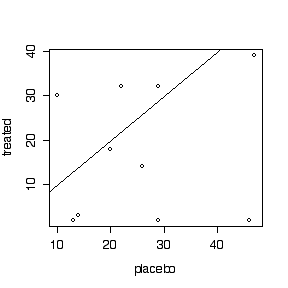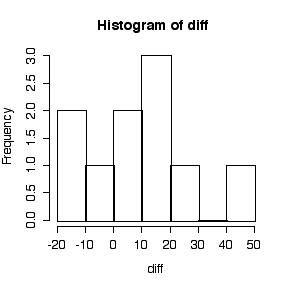• Independence: can't test.
• Normality: considering the small sample size, the histogram (or box plot or dot plot or stem and leaf plot) is reasonably symmetric and free of outliers, so the assumption of normality is not unreasonable.
• Constant mean difference: the points at the right of the scatter plot tend to lie further below the diagonal than the points at the left, suggesting that the magnitude of the difference increases with the "Before" level, so an analysis of ratios or differences in logs, rather than arithmetic differences, is suggested but not required.

Test the hypothesis that the mean difference is zero:

```> dbar <- mean(diff)
> sd <- sqrt(var(diff))
> dbar
 8.2
> sd
 18.12181
> sd^2
 328.4
> t0 <- (dbar-0)/(sd/sqrt(10))
> t0
 1.43091
> 2*(1-pt(t0,9))
 0.1862470
```

Since t0 = 1.430 is in the right tail of the t distribution on 9 degrees of freedom, using the tables in Rosner, t0 lies between t9,0.90 = 1.383 and t9,0.95 = 1.833 so we have that 0.2 > P > 0.1. (The exact value is P = 0.186.)

Because the P-value is large, we conclude that there is no evidence from these data that the cream is any more or less effective than the placebo in reducing muscle soreness.

#### Q3

Suppose that you are going to repeat the study described in 2(b) but this time you want the confidence interval for the mean difference to be ± 3 units. How many patients will you need? What would be achieved by doing the larger study? [5 marks]

Taking sd = 18.12181 and t0.025, n-1 = 2 as the best available values, solve for n in t0.025, n-1 * sd / sqrt(n) = 3 to get

n = (2 * 18.12181 /3)2 = 146 patients.

The larger study would be worth doing because the results of the present study suggested that the cream might be effective: several patients had much reduced soreness, but other patients had little or no reduction or even increased soreness, and hence because of the small sample size, if the cream was effective, the effect was lost in the variation. A larger study would estimate the effectiveness of the cream more accurately and could detect a much smaller effect than this study could.